# How To Return Sheet Name In A Cell

Feb 03, 2020 • edited Feb 04, 2020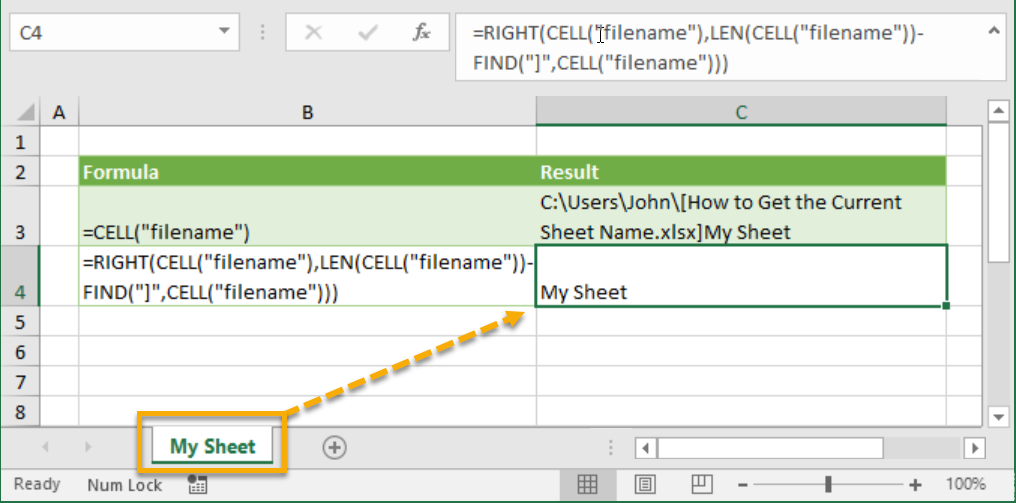In this article, we will learn how to get sheet name only in Excel.

Return the name of a sheet into a cell using an Excel formula. This video tutorial explores the use of the CELL, MID and FIND functions to display the worksheet name in a cell.

# Get Sheet Name

To return the sheet name in a cell, use the CELL, FIND and the MID function in Excel. There's no built-in function in Excel that can get the sheet name.

1. The CELL function below returns the complete path, workbook name and current worksheet name.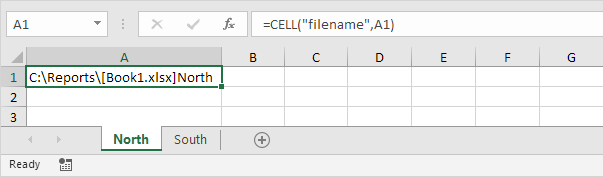Note: instead of using A1, you can refer to any cell on the first worksheet to get the name of this worksheet.

2. Use the FIND function to find the position of the right bracket. Add 1 to return the start position of the sheet name.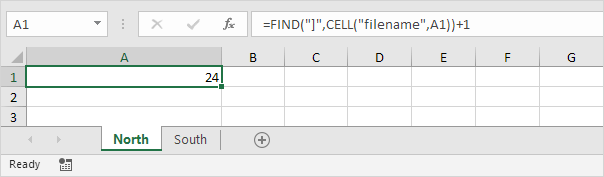3. To extract a substring, starting in the middle of a string, use the MID function. First argument (formula from step 1). Second argument (formula from step 2). Third argument (31).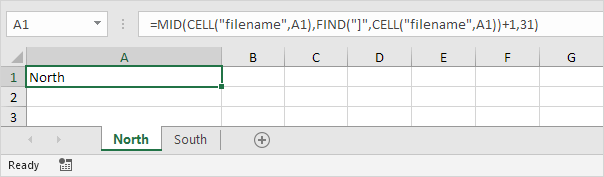Explanation: the MID function shown above starts at position 24 and extracts 31 characters (maximum length of a worksheet name).

4. To get the name of the second worksheet, simply refer to any cell on the second worksheet.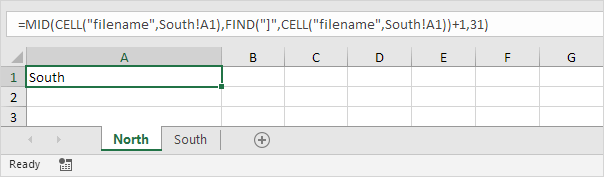#How To#Tutorial#Worksheets

How To Show Fewer Decimal Places in Excel

How To View Multiple Excel Worksheets